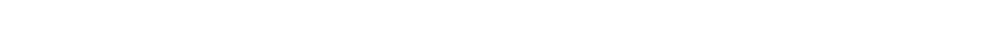#si',\$content,\$matches); //var_dump(\$matches); foreach (\$matches as \$key => \$p) { if(preg_match('/color:\s*#333399;/si',\$p)){ \$conArr[]['tit'] = preg_replace('/<[^<]+?>/si', '', \$p); }else{ if(count(\$conArr) != 0){ \$conArr[count(\$conArr)-1]['step'] .= \$p; } } } //var_dump(\$conArr); return \$conArr; } \$cats = array_merge(subcategory(\$catid)); foreach(\$cats as \$k => \$cat){ \$cats[\$k]['sub'] = array_merge(subcategory(\$cat['catid'])); } foreach (\$cats as \$k => \$cat) { foreach (\$cat['sub'] as \$i => \$subcat) { if(preg_match('/\d+\$/',\$subcat['abbr'],\$match)){ \$cats[\$k]['sub'][\$i]['steps'] = getContent(\$match); } } } //var_dump(\$cats); ?>

# 深圳之窗

## 办理地点

深圳随迁入户办理须知：

被投靠人户籍地公安分局或派出所户政窗口(其中被投靠人户籍在罗湖、福田、南山、盐田四区及光明、坪山、龙华、大鹏新区的，到被投靠人户籍所在区(新区)公安分局人口管理科(治安大队)申请;被投靠人户籍在宝安、龙岗两区及龙华新区的，到被投靠人户籍所在地派出所申请)。

## 常见问题